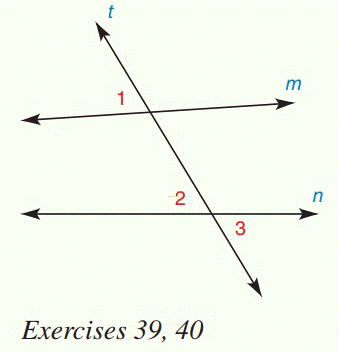Chapter 2.CR, Problem 39CR### Elementary Geometry for College St...

6th Edition
Daniel C. Alexander + 1 other
ISBN: 9781285195698

#### Solutions

Chapter
Section### Elementary Geometry for College St...

6th Edition
Daniel C. Alexander + 1 other
ISBN: 9781285195698
Textbook Problem
1 views

# Given: m ∥ n Prove: ∠ 1 ≅ ∠ 2To determine

To prove:

1 is not congruent to 2.

Explanation

Given:

Line m is not parallel to n.

Figure (1)

Concept:

If two lines are cut by a transversal so that a pair of corresponding angles is congruent, then these lines are parallel.

Approach:

Line m is not parallel to n.

Assume 12

So,

### Still sussing out bartleby?

Check out a sample textbook solution.

See a sample solution

#### The Solution to Your Study Problems

Bartleby provides explanations to thousands of textbook problems written by our experts, many with advanced degrees!

Get Started

#### Evaluate the integrals in Problems 1-32. Identify the formula used. 19.

Mathematical Applications for the Management, Life, and Social Sciences

#### Compute P5,2.

Understanding Basic Statistics

#### The directrix of the conic given by is:

Study Guide for Stewart's Multivariable Calculus, 8th

#### True or False: x2x24dx should be evaluated using integration by parts.

Study Guide for Stewart's Single Variable Calculus: Early Transcendentals, 8th